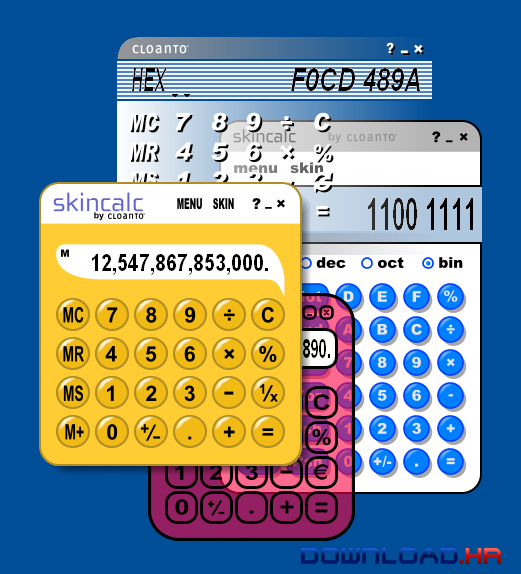Top 10 Calculators for Windows OS in 2020
Marko Jovanovic Feb 6th, 2020
Check our list of the top 10 Calculators software products in 2020If you’re here reading this, chances are you’re trying to find the best calculators software products for Windows OS. Finding the right program isn't an easy job because of the variety of software available out there. Some of them are free and some of them are paid bringing additional features to users. So, in order to help you here is the list of the 10 best calculators software products you can try rated by number of user downloads on Download.io. Hope that you will find our list useful and that you will find the right software for you!

### GeoGebra

Dynamic mathematics software used for geometry, algebra and calculus
GeoGebra is a very useful  mathematics tool for education in secondary schools, which brings together geometry, algebra and calculus. GeoGebra is also a dynamic geometry system, meaning you can do constructions with points, segments, vectors, lines, conic sections as well as functions and change them dynamically afterwards. On the other hand, equations and coordinates can be entered directly. Thus, GeoGebra has the ability to deal with variables for numbers, vectors and points, finds derivatives and integrals of functions and offers commands like Root or Extremum. These two views are characteristic of GeoGebra: an expression in the algebra window corresponds to an object in the geometry window and vice versa.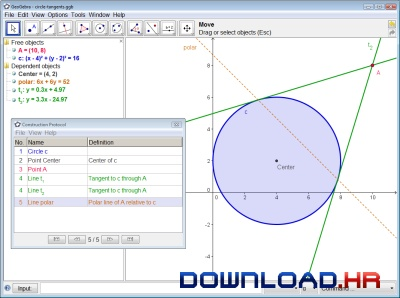### Free Scientific Calculator CJ47E

CJ47E - Free Scientific calculator for Windows with complex numbers
CJ47E - Free Scientific calculator for Windows with complex numbers
- Logarithms and exponentials.
- Fractions and root fractions output format
- Polar and binomic complex numbers output format
- Trigonometric functions
- Rectangular-polar conversion.
- Scientific Constants
- 24 user's variables...
- User's Manual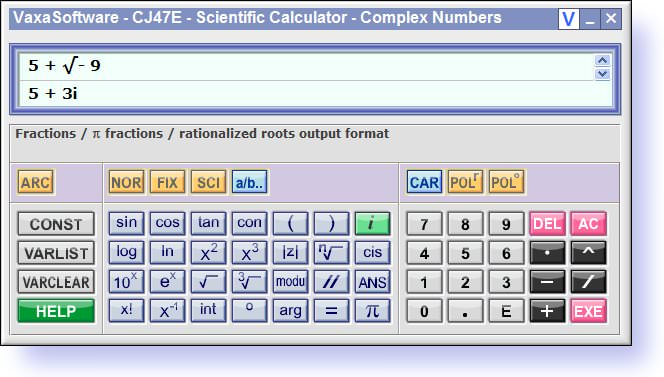### Equalizer

Equalizer is a freeware scientific expression calculator
Equalizer has a simple text line on which you can write the expression in the same way you'd write it on paper (expression calculator: typing '(6*5-12) / 2' will immediately show the answer: '9'). Also Includes a Graph plotter.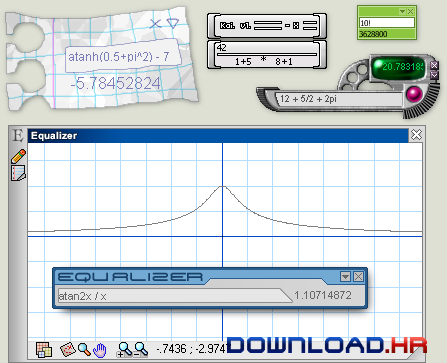### Calculator Prompter

Calculator Prompter is a math expression calculator
Calcualtor Prompter has a built-in error recognition system that helps you get correct results. With Calculator Prompter you can enter the whole expression, including brackets, and operators. You can use Calculator to perform any of the standard operations for which you would normally use a handheld calculator. Calculator performs arithmetic, such as root (#) and power (^).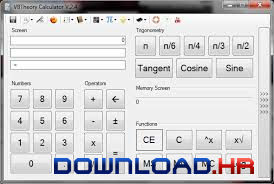### RedCrab

The calculator with fullscreen editor
RedCrab is a highly efficient calculator that will enable users to work in fullscreen mode. The mathematical expressions are not typed in a typical command line, but can be enter in the editor window. RedCrab display the result either next to the expression or such as in any position in engineering drawings. The handling is easier than conventional calculators. RedCrab supports the entry of complex algebraic equations with fractions, square roots, exponents, etc. for technical and scientific applications. Several mathematical functions can be written on the same page. Results from one expression can be carried over to the next task. You can save and load the formulas to create a formula library. Complex tasks can be explained visually by importing drawings in bitmap or JPEG format.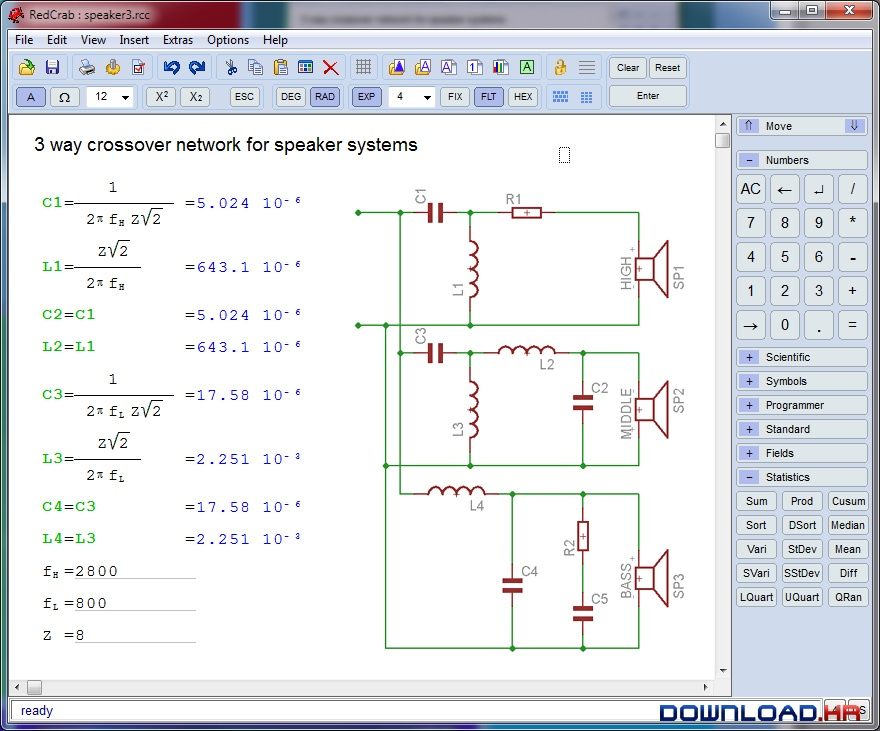### Metalogic Calculator

This calculator allows natural entry of equation
This calculator allows natural entry of equations (i.e. 17-(9*8) - 7 + 34.23). Then it goes beyond just giving you a result, the "steps" box, shows you how it solved the equation step by step. Results are given simultaneously in dec/hex/oct/bin.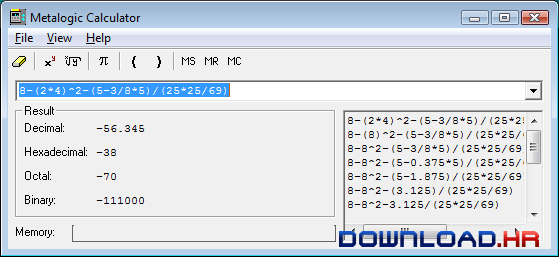### Precise Calculator

High precision scientific calculator for Windows.
Precise Calculator has more than 150 mathematical functions and all of them can return as many digits as you need. It can calculate with integers, real numbers, complex numbers, fractions, vectors, matrices. Commands if, goto, for, print can be used to create scripts. You can use history to edit and repeat previous expressions. Frequently used expressions can be saved as macro.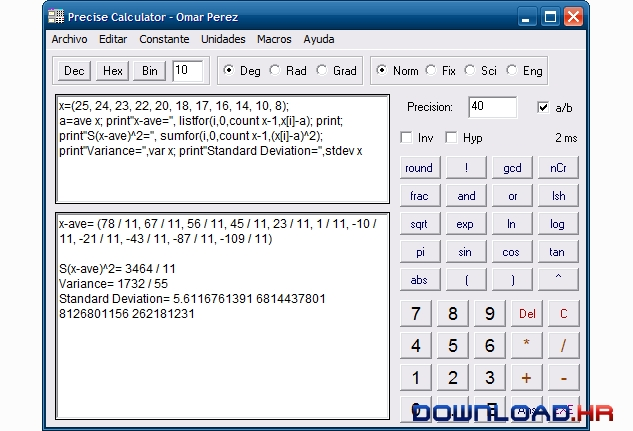### ESBCalc

ESBCalc is a Freeware Win32 Scientific Calculator with Infix Notation, Brackets, Scientific Functions, Memory, Optional Paper Trail, Result History List, Customisation of the Colours & Fonts used, Customisation of the Hints, and more. Functions include Trigonometric, Hyperbolic, Logarithmic - Base 10, Base 2 & Natural - and many more.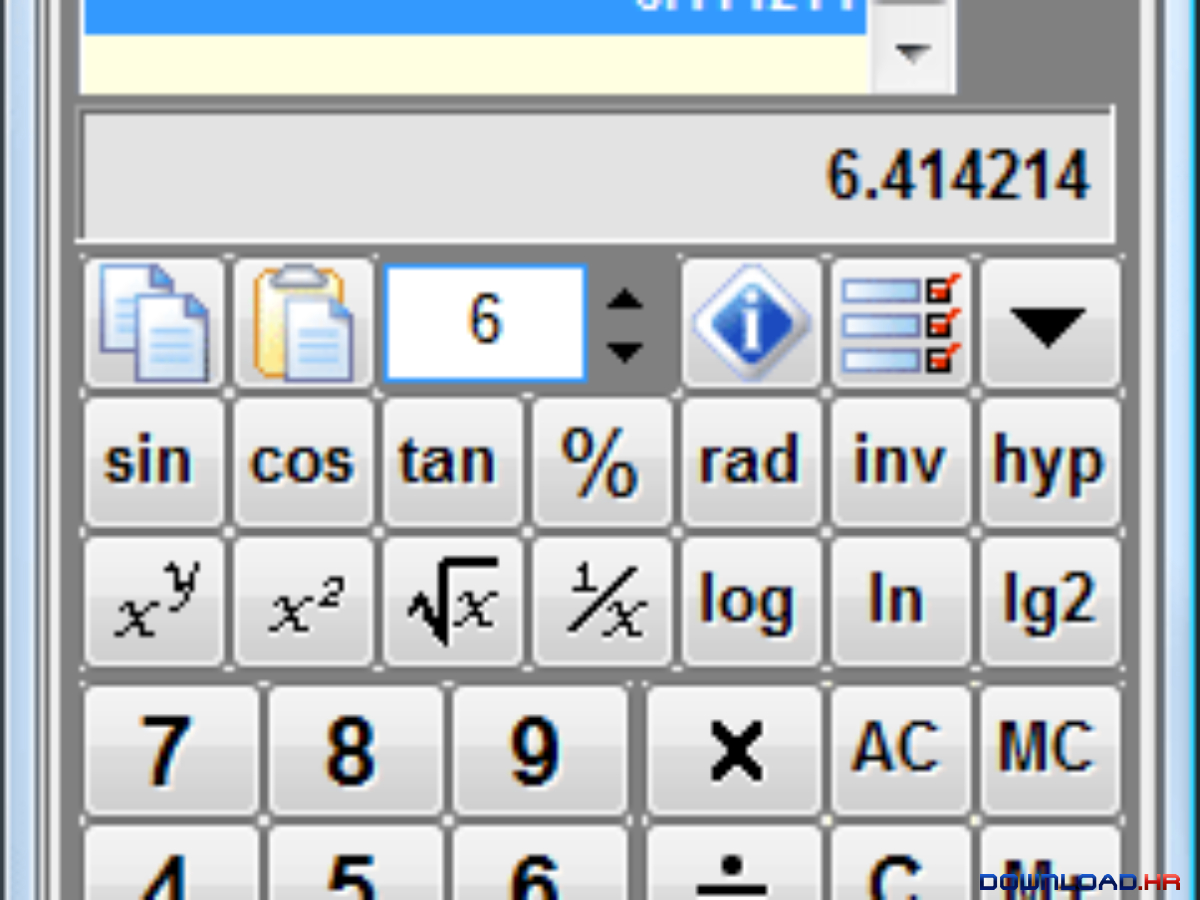### Karen's Calculator

Karen's Calculator is a high-precision calculator
Karen's Calculator is a high-precision calculator. Unlike ordinary calculators, it returns completely accurate results, even with operands containing thousands of digits. If you're patient, operands and results containing hundreds of thousands of digits are possble too!

Most calculators are only accurate when dealing with numbers containing a few digits -- usually 16 to 32. When asked to calculate using larger numbers, they either fail (displaying an error) or return results that are only approximately accurate.

Karen's Calculator can easily handle numbers and results containing many thousands of digits with complete accuracy. It also includes an on-screen "tape" -- which can be saved to disk -- versatile memory features, and more!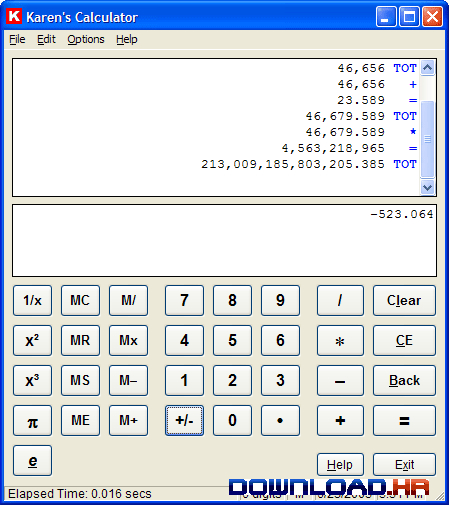### SkinCalc

SkinCalc, which is completely free, features standard and advanced calculator functionality
SkinCalc, which is completely free, features standard and advanced calculator functionality, a spreadsheet interface, and skins. Skins make it possible to change the layout and functionality of the software, with effects that include non-rectangular shapes, user-configurable transparency and alpha channel translucency. More than 100 skins are available from the sites listed at homepage, which also has instructions to create your own skins.

Calculator Functions
The calculator has a variety of built-in functions which can be used to configure the user interface for custom needs.

The following functions are available in the default configuration (as in the "SkinCalc" and "SkinCalc Pro" skins): Plus, Minus, Multiply, Divide, Decimal, Change Sign, Equals, Percent, Square (by pressing "Multiply Equals"), Square Root ("Divide Equals"), Memory Clear/Recall/Set/Add, Clear, Clear All ( "Clear Clear"), Backspace and drop-down menu with list of units. This configuration was designed to offer at least the full functionality of the Windows system calculator (Standard Mode).

Skin files can be used to change the layout and to add/remove functionality. Additional keys that can be programmed include: Multiply and divide by Constant (very useful for adding or removing VAT), Double-Zero, Triple-Zero, Reciprocal ("1/x"), Square, Square Root, Base Conversion, Bitwise Operators, Clear All, Convert to Previously-Used Unit and Convert to Preset Unit. More advanced features include support for advertising banners, dual display, skin selection menu, button shortcuts to access the Skins tab and other software options.